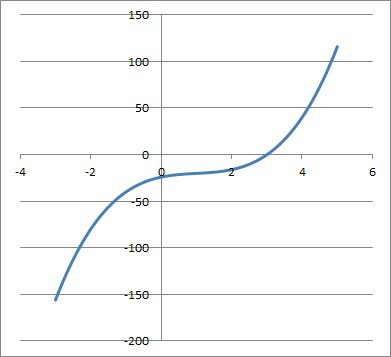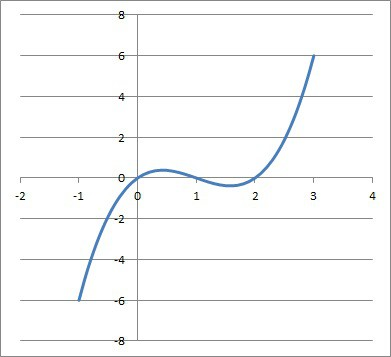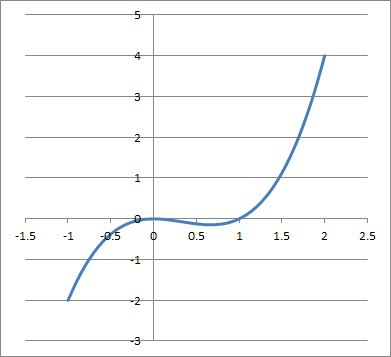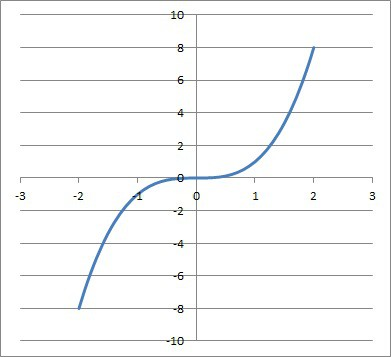# Solutions Of Cubic Functions (3 Key Facts About Zeros Of Cubics)

Cubic functions are not seen as commonly as quadratics, but they are still important in mathematics.  We can say a lot about the roots of cubic functions and what they look like (real or complex, and how many of each).

So, how many real solutions does a cubic function have?  A cubic function with real coefficients has at least one real root, since complex roots come in conjugate pairs.  A cubic function can have 1 real root (repeated 3 times, or 1 real root and 2 complex roots), 2 real roots (when one real root is repeated twice), or 3 distinct real roots.

Of course, a cubic always has at least 1 real root – if we can find it, then we can factor the cubic into the product of a linear function and a quadratic function.  We can then use the quadratic formula to find the other two roots easily.

In this article, we’ll talk about the roots (solutions) of cubic functions and when they will be real or complex.  We’ll also answer some common questions about the roots of cubic functions.

Let’s begin.

Having math trouble?

Looking for a tutor?

## Solutions Of Cubic Functions

There are several different cases for the solutions of cubic functions, depending on how many real and complex roots there are in the equation:

• One Triple Real Root – this happens when there is only one real root and no complex roots.  The real root is repeated three times.  The simplest example is f(x) = x3, which factors as x*x*x, with a triple real root of x = 0.
• One Real Root & Two Complex Conjugate Roots – this is the only case where there are complex roots.  This happens when there is a real root (not repeated) and two complex conjugate roots.  In this case, the cubic will factor as f(x) = k(x – r)(x – z1)(x – z2), where k is a constant, r is the real root, z1 = a + bi is one complex root, and z2 = a – bi is the other complex root.  Note that z1 and z2 are complex conjugates, meaning they have the same real part but opposite imaginary parts.
• Two Real Roots (One Double Real Root) – this happens when there is a double real root and a 2nd real root (not repeated).  One example is f(x) = x3 – x2, which factors as x*x*(x – 1), with a double real root of x = 0 and a real root of x = 1 (not repeated).
• Three Distinct Real Roots – this happens when there are 3 different real roots of the cubic function.  One example is f(x) = x3 – 3x2 + 2x, which factors as x(x – 1)(x – 2), with real roots x = 0, x = 1, and x = 2.

The table below summarizes the four cases for the zeros of a cubic and how many roots are real or complex.

Remember that a cubic always has at least one real root.  This allows us to factor the cubic as the product of a linear function and a quadratic function.

There are 3 possible cases for the solutions of a quadratic function:

If we are in Case 1 and the root of the linear function is distinct from both real roots of the quadratic, then we get three distinct real roots.

If we are in Case 1 and the root of the linear function is the same as one of the real roots of the quadratic, then we get two distinct real roots (one of them is a double root).

If we are in Case 2 and the root of the linear function is the same as the repeated root of the quadratic, then we get one triple real root.

If we are in Case 2 and the root of the linear function is distinct from the repeated root of the quadratic, then we get then we get two distinct real roots (one of them is a double root).

If we are in Case 3, then we get one real root and two complex conjugate roots.

The table below summarizes the cases for the nature of the roots of a cubic function, depending on the roots of the quadratic and the linear factor.

### How Many Zeros Does A Cubic Function Have?

A cubic function has 3 zeros, counting repeated roots.  However, some of them may be repeated (a double or triple root is possible), or there be a pair of complex (non-real) roots.

According to the Fundamental Theorem of Algebra, a polynomial of degree n with real coefficients has n complex roots (counting repeated roots).  Applying this to a cubic with real coefficients (n = 3), we can see that such a function has 3 roots.

### Can A Cubic Function Have Imaginary Roots?

A cubic function can have imaginary roots in some cases.  A cubic function will always have either 0 or 2 imaginary roots, which must be complex conjugates of one another (according to the Complex Conjugate Root Theorem).

For example, if x = 2i is a root of a cubic f(x), then x = -2i (the complex conjugate of 2i) is also a root of f(x).  The third root of this cubic would be real – let’s say x = 3.

Then our cubic f(x) would look like this:

• f(x) = k(x – 3)(x – 2i)(x + 2i)
• f(x) = k(x – 3)(x2 + 2ix – 2ix – 4i2)  [FOIL (x – 2i)(x + 2i)]
• f(x) = k(x – 3)(x2 + 4)  [cancel like terms and use i2 = -1]
• f(x) = k(x3 + 4x – 3x2 – 12) [FOIL]

If we know the coordinates of one point on the cubic f(x) that is not a zero, then we can find the value of k.  Let’s say we have the point (1, -20) on the graph of f(x).

Then we can solve for k as follows:

• f(1) = k(13 + 4(1) – 3(1)2 – 12)  [substitute x = 1]
• -20 = k(1 + 4 – 3 – 12)  [f(1) = -20, since the point (1, -20) is on the graph of f(x)]
• -20 = k(-10)
• 2 = k

Since k = 2, we can write out the entire function as:

• f(x) = k(x3 + 4x – 3x2 – 12)
• f(x) = 2(x3 + 4x – 3x2 – 12) [k = 2]
• f(x) = 2x3 – 6x2 + 8x – 24 [distribute 2 through parentheses]

The graph of the function is pictured below.The graph of the cubic function f(x) = 2x3 – 6x2 + 8x – 24. We can see one zero at x = 3, and the other two are complex: 2i and -2i.

Having math trouble?

Looking for a tutor?

### Can A Cubic Function Have No Real Zeros?

A cubic function always has at least 1 real zero, due to the Fundamental Theorem of Algebra and the Complex Conjugate Root Theorem.

The Fundamental Theorem of Algebra tells us that a cubic has exactly 3 roots.  The Complex Conjugate Root Theorem tells us that complex roots must come in pairs (which are complex conjugates).

So, the possible cases are:

• 2 complex roots (a complex conjugate pair) and 1 real root
• 0 complex roots and 3 real roots (either 3 distinct real roots or 2 real roots, one of which is a double root)

You can see an example of the 2nd case (3 distinct real roots) below.The graph of the cubic function f(x) = x3 – 3x2 + 2x, which has 3 distinct real roots: x = 0, x = 1, and x = 2.

If the cubic had no real roots, then there would be 3 complex roots, one of which would not be paired with its complex conjugate.

The Complex Conjugate Root Theorem implies that a cubic function can never have 1 or 3 imaginary roots (a cubic function can only have 0 or 2 complex roots).  It also means a cubic function must have at least one real root.

### Can A Cubic Function Have 3 Imaginary Roots?

A cubic function with real coefficients cannot have 3 imaginary roots, since this would imply no real roots, which we showed was impossible above.

As mentioned earlier, a cubic function with real roots has either 0 or 2 complex roots, since the complex roots come in conjugate pairs by the Complex Conjugate Root Theorem.

The only way a cubic function can have 3 imaginary roots is if the coefficients are complex (instead of restricted to real coefficients).

### Can A Cubic Function Have A Double Root?

A cubic function can have a double root.  However, this double root must be real.

One example is the cubic function f(x) = x3 – x2.  This function factors as x*x*(x – 1), with a double real root of x = 0 (repeated) and a real root of x = 1 (not repeated).

The graph is pictured below.The the cubic function f(x) = x3 – x2, which has a double real root at x = 0 and another real root at x = 1 (not repeated).

If the double root were complex, then its complex conjugate would also need to be a double root (by the Complex Conjugate Root Theorem).

However, this would imply at least 4 zeros of the function, contradicting the Fundamental Theorem of Algebra (which states that a cubic has 3 roots).

### Can A Cubic Function Have 2 Zeros?

A cubic function can have 2 zeros if one of them is a repeated real root (double root).  This implies that there will be no complex roots (no complex conjugate pair).

In this scenario, there will be one double real root (that is repeated) and one real root (that is not repeated).

One example is the cubic function f(x) = x3 – x2.  This function factors as x*x*(x – 1), with a double real root of x = 0 (repeated) and a real root of x = 1 (not repeated).

### Can A Cubic Function Have Only One Real Root?

A cubic function can have only one real root.  There are two cases where this can happen:

• One Triple Real Root – the simplest example is f(x) = x3, which factors as x*x*x, with a triple real root of x = 0.
• One Real Root & Two Complex Conjugate Roots – one example is f(x) = x3 + x, which factors as x(x + i)(x – i), with a real root of x = 0 and complex conjugate root pair x = i and x = -i.

The graph of the first case is pictured below.The graph of the cubic function f(x) = x3, which has a triple real root at x = 0. There are no complex roots.

## Conclusion

Now you know about the solutions of cubic functions and when they are real or complex.  You also know that complex roots come in conjugate pairs for polynomials with real coefficients, whether they are quadratic, cubic, or higher order polynomials.